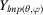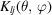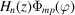International Tables for Crystallography (2006). Vol. C. ch. 6.1, pp. 554-595https://doi.org/10.1107/97809553602060000600

## Contents

• 6.1. Intensity of diffracted intensities  (pp. 554-595)
• 6.1.1. X-ray scattering  (pp. 554-590) | html | pdf |
• 6.1.1.1. Coherent (Rayleigh) scattering   (p. 554) | html | pdf |
• 6.1.1.2. Incoherent (Compton) scattering  (p. 554) | html | pdf |
• 6.1.1.3. Atomic scattering factor  (pp. 554-565) | html | pdf |
• 6.1.1.3.1. Scattering-factor interpolation  (p. 565) | html | pdf |
• 6.1.1.4. Generalized scattering factors  (pp. 565-584) | html | pdf |
• 6.1.1.5. The temperature factor  (pp. 584-585) | html | pdf |
• 6.1.1.6. The generalized temperature factor  (pp. 585-590) | html | pdf |
• 6.1.1.6.1. Gram–Charlier series  (p. 586) | html | pdf |
• 6.1.1.6.2. Fourier-invariant expansions  (pp. 586-588) | html | pdf |
• 6.1.1.6.3. Cumulant expansion  (p. 588) | html | pdf |
• 6.1.1.6.4. Curvilinear density functions  (pp. 588-589) | html | pdf |
• 6.1.1.6.5. Model-based curvilinear density functions  (pp. 589-590) | html | pdf |
• 6.1.1.6.6. The quasi-Gaussian approximation for curvilinear motion  (p. 590) | html | pdf |
• 6.1.1.7. Structure factor  (p. 590) | html | pdf |
• 6.1.1.8. Reflecting power of a crystal  (p. 590) | html | pdf |
• 6.1.2. Magnetic scattering of neutrons  (pp. 590-593) | html | pdf |
• 6.1.2.1. Glossary of symbols  (pp. 590-591) | html | pdf |
• 6.1.2.2. General formulae for the magnetic cross section  (p. 591) | html | pdf |
• 6.1.2.3. Calculation of magnetic structure factors and cross sections  (p. 591) | html | pdf |
• 6.1.2.4. The magnetic form factor  (p. 592) | html | pdf |
• 6.1.2.5. The scattering cross section for polarized neutrons  (pp. 592-593) | html | pdf |
• 6.1.2.6. Rotation of the polarization of the scattered neutrons   (p. 593) | html | pdf |
• 6.1.3. Nuclear scattering of neutrons  (pp. 593-595) | html | pdf |
• 6.1.3.1. Glossary of symbols  (p. 593) | html | pdf |
• 6.1.3.2. Scattering by a single nucleus  (pp. 593-594) | html | pdf |
• 6.1.3.3. Scattering by a single atom  (p. 594) | html | pdf |
• 6.1.3.4. Scattering by a single crystal  (pp. 594-595) | html | pdf |
• References | html | pdf |
• Figures
• Fig. 6.1.1.1. Scattering by an electron  (p. 554) | html | pdf |
• Fig. 6.1.2.1. The integrals < j 0 >, < j 2 >, and < j 4 > for the Fe 2+ ion plotted against(p. 592) | html | pdf |
• Fig. 6.1.2.2. Comparison of 3 d , 4 d , 4 f , and 5 f form factors  (p. 592) | html | pdf |
• Fig. 6.1.3.1. Dependence on neutron wavelength of the coherent scattering length of 113 Cd  (p. 594) | html | pdf |
• Tables
• Table 6.1.1.1. Mean atomic scattering factors in electrons for free atoms  (pp. 555-564) | html | pdf |
• Table 6.1.1.2. Spherical bonded hydrogen-atom scattering factors  (p. 565) | html | pdf |
• Table 6.1.1.3. Mean atomic scattering factors in electrons for chemically significant ions  (pp. 566-577) | html | pdf |
• Table 6.1.1.4. Coefficients for analytical approximation to the scattering factors of Tables 6.1.1.1 and 6.1.1.3  (pp. 578-580) | html | pdf |
• Table 6.1.1.5. Coefficients for analytical approximation to the scattering factors of Table 6.1.1.1 for the range 2.0 < (sin)/λ < 6.0 Å −1 [equation (6.1.1.16)]  (p. 581) | html | pdf |
• Table 6.1.1.6. Angle dependence of multipole functions, normalized as in equation (6.1.1.23); ω = cosand S , D , Q , O , H denote scalar, dipole, quadrupole, octupole, and hexadecapole terms, respectively   (p. 583) | html | pdf |
• Table 6.1.1.7. Indices allowed by the site symmetry for the real form of the spherical harmonics(p. 584) | html | pdf |
• Table 6.1.1.8. Cubic harmonicsfor cubic site symmetries  (p. 585) | html | pdf |
• Table 6.1.1.9. f nl (α, S ) = ∫ 0 r n exp(−α r ) j l ( Sr ) d r   (p. 586) | html | pdf |
• Table 6.1.1.10. Indices nmp allowed by the site symmetry for the functions(p. 586) | html | pdf |
• Table 6.1.1.11. Indices n x , n y , n z allowed for the basis functions H n x ( Ax ) H n y ( By ) H n z ( Cz )  (p. 587) | html | pdf |